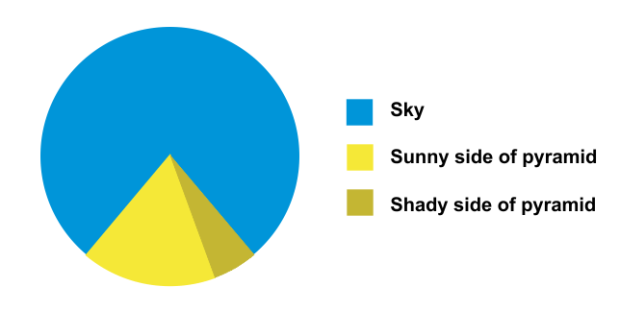# Infographic

Probability Level 1Consider the pie chart shown above, where the ratio of angles formed by the 3 sectors are $3 : 14 : 1$. In degrees, what is the measure of angle formed for the sector that represents the shady side of the pyramid?

×